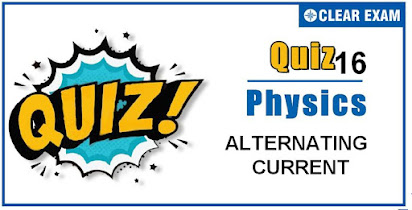## [LATEST]\$type=sticky\$show=home\$rm=0\$va=0\$count=4\$va=0

As per analysis for previous years, it has been observed that students preparing for NEET find Physics out of all the sections to be complex to handle and the majority of them are not able to comprehend the reason behind it. This problem arises especially because these aspirants appearing for the examination are more inclined to have a keen interest in Biology due to their medical background. Furthermore, sections such as Physics are dominantly based on theories, laws, numerical in comparison to a section of Biology which is more of fact-based, life sciences, and includes substantial explanations. By using the table given below, you easily and directly access to the topics and respective links of MCQs. Moreover, to make learning smooth and efficient, all the questions come with their supportive solutions to make utilization of time even more productive. Students will be covered for all their studies as the topics are available from basics to even the most advanced. .

Q1. A bulb is connected first with dc and then ac of same voltage it will shine brightly with
•  AC
•  DC
•  Brightness will be in ratio 1/1.4
•  Equally with both
Solution
Brightness ∝Pconsumed∝1/R. For bulb, Rac=Rdc, so brightness will be equal in both the cases

Q2. In an ac circuit, the r.m.s. value of current, Irms is related to the peak current, I0 by the relation
•  Irms=1/π I0
•  Irms=1/√2 I0
•  Irms=√2 I0
•  Irms=πI0
Solution
It is a fact.

•  2A
•  2.4 A
•  Zero
•  1.7 A
Solution
Given XL=XC=5Ω, this is the condition of resonance. So VL=VC, so net voltage across L and C combination will be zero

Q4. A fully charged capacitor C with initial charge q0 is connected to a coil of self inductance L at t = 0. The time at which the energy is stored equally between the electric and the magnetic fields is
•  π/4 √LC
•  2π√LC
•  √LC
•  π√LC
Solution
As initially charge is maximum, q=q0cos⁡ωt

Q5. The phase difference between the current and voltage of LCR circuit in series combination at resonance is
•  O
•  π/2
•  π
•
Solution
At resonance LCR series circuit behaves as pure resistive circuit. For resistive circuit ϕ=0°

Q6. In an L – C – R series AC circuit, the voltage across each of the components. L, C and R is 50 V. The voltage across the L – C combination will be
•  50 V
•  50√2 V
• 100 V
•  zero
Solution
In an L – C – R series AC circuit, the voltage across inductor L leads the current by 90° and the voltage across capacitor C lags behind the current by 90°.
Hence, the voltage across L-C combination will be zero.

Q7. In an induction coil, the coefficient of mutual inductance is 4H. If current of 5A in the primary coil is cut off i 1/ 1500s, the emf at the terminals of the secondary coil will be
•  15 kV
•  60 kV
•  10 kV
•  30 kV
Solution
e=Ldi/dt = 4 ×5/(1/1500)=30000V=30kV

Q8. The maximum value of AC voltage in a circuit is 707 V. Its rms value is
•  70.7 V
•  100 V
•  500 V
•  707 V
Solution
Vrms=V0/√2=(770√2)/2≈500 V

Q9. A metal rod of resistance 20 Ω is fixed along a diameter of a conducting ring of radius 0.1 m and lies on x-y plane. There is a magnetic field B ⃗ = (50 T) k ̂. The ring rotates with an angular velocity ω=20 rads-1 about its axis. An external resistance of 10 Ω is connected across the centre of the ring and rim. The current through external resistance is
•  1/2A
•  1/3A
•  1/4A
•  zero
Solution
Here, resistance of rod =2Ω.r=0.1 m,B=50 T,along z-axis ω=20 rads-1. Potential difference between centre of the ring and the rim is V=1/2 Bωr2=1/2 ×50×20×0.12=5 V The equivalent circuit of the arrangement is shown in figure

Q10.  An ac source of variable frequency f is connected to an LCR series circuit. Which of the graphs in figure represents the variation of current I in the circuit with frequency f
Solution
As explained in solution (1) for frequency 0-fr,Z decreases hence (i=V/Z) increases and for frequency fr-∞,Z increases hence i decreases## Want to know more

Please fill in the details below:

## Latest NEET Articles\$type=three\$c=3\$author=hide\$comment=hide\$rm=hide\$date=hide\$snippet=hide

Name

ltr
item
BEST NEET COACHING CENTER | BEST IIT JEE COACHING INSTITUTE | BEST NEET & IIT JEE COACHING: Quiz-16 ALTERNATING CURRENT
Quiz-16 ALTERNATING CURRENT